# Why Alpha Works - And A New Way Of Calculating It

|
Includes:
by: Yuval Taylor
Summary

Explains the concepts of alpha and beta in layman’s terms.

Describes alpha’s advantages over other performance measures.

Explains why alpha and beta are inversely correlated and why low-beta strategies outperform.

Proposes a new way to calculate alpha that is not based on least squares estimation.

## What Is Alpha?

Alpha has, over the last 50 years, become the standard way to measure the active return of a portfolio: how much the portfolio outperformed the benchmark.

Technically, however, it's a point on a line, specifically the point where the line crosses the y axis.

And that line is the linear regression line between the portfolio's returns minus the risk-free rate (on the y axis) and the benchmark's returns minus the risk-free rate (on the x axis). In other words, you make a graph out of a lot of dots. Each dot has the benchmark's return minus the risk-free rate as its x value and your return minus the risk-free rate as its y value. You then find the straight line that best fits all the dots, and that's your linear regression line. Where it crosses the y axis is your alpha, and the slope of the line is your beta.

When beta is high (above one), the portfolio's returns vary a great deal proportionally to those of the benchmark. When beta is low (below one), either the portfolio's returns and the benchmark's are relatively uncorrelated or the variation in the portfolio's returns is significantly less than that of the market's. When beta is negative, the portfolio's returns move opposite to those of the benchmark.

As for alpha, to put it plainly, it's the expected return of the portfolio when the benchmark's return equals the risk-free rate.

## Why Use Alpha as a Performance Measure?

The most obvious performance measure is simple return: the amount in the portfolio at the end of a certain time period divided by the amount at the beginning, minus one. However, this measure doesn't take into account what the rest of the market is doing, and it's entirely dependent on the beginning and ending dates. It's a more or less meaningless measure.

A more meaningful measure would be less dependent on beginning and ending dates and would take into account the movements of the market. There are various such measures besides alpha. Average excess return, median excess return, and the information ratio are three of them. All of them rely on simple subtraction: one subtracts the monthly (or weekly) market returns from the portfolio returns to obtain the excess returns. You then take either the average, the median, or the average divided by the deviation from the average for these respective measures.

Subtraction, however, is less sophisticated and less accurate a tool than regression. A regression line has the form y = α + βx, while subtraction is simply y = x + e (where y is the portfolio return, x is the market return, and e is the excess return). Subtraction is essentially the same as regression except that β is always equal to one. If you actually look at the data, β often does not come close to one.

Let's look, for example, at a scatter plot of the last five years of monthly returns of the SPDR S&P Oil & Gas Equipment & Services ETF (NYSEARCA:XES) compared to the returns of the S&P 500. (Please note that from now on, for the purposes of keeping this article relatively simple, I will not be subtracting the risk-free rate from monthly returns). The percentage returns of XES are plotted along the y axis while the returns of the S&P 500 are plotted along the x axis. The orange dotted line is the line y = x, or β = 1.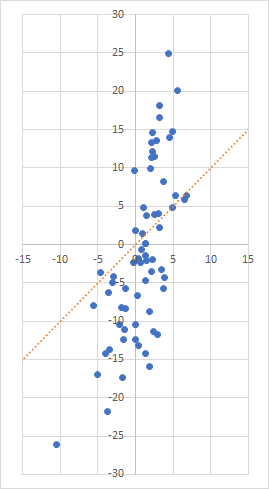Using only excess returns would mean either using the orange line to describe the data or moving it wholesale up or down, keeping its slope the same. You wouldn't be able to tilt that line at all. In other words, the line doesn't fit the data very well, even if you move it down a few percentages.

That's why alpha and beta, for many money managers, are the ideal numbers for characterizing portfolio returns. Those two numbers capture the relationship of the portfolio to the benchmark better than any others I've come across.

## Alpha, Beta, and Risk

Shortly after alpha and beta were introduced to the investment world in the 1960s, it was discovered that alpha and beta were inversely correlated, no matter what asset class you looked at.

The inverse correlation of alpha and beta is inherent in the mathematical relationship of the two variables as long as the market's returns are more likely to be positive than negative. Remember that the equation y = α + βx can be rewritten as α = y ­- βx. It's clear from this that if you have two strategies with the same return (x and y are the same), and if the adjusted market return (x) is greater than zero, the strategy with the higher beta will have the lower alpha; it's also clear that most investable asset classes have market returns which are greater than zero more frequently than they are less than zero. In fact, I have proven mathematically that alpha and beta are indeed inversely correlated when market returns are usually greater than zero. But this idea did not occur to the scholars who came up with the ideas of applying linear regression to finance, and instead they have spent close to 50 years trying to come up with behavioral reasons for the inverse correlation.

Portfolio returns tend to correlate with alpha far better than they correlate with beta. Look again at the equation y = α + βx. Because x (the market's return) is sometimes negative or zero, a positive correlation of y and α is going to be far more frequent than a positive correlation of y and β. In fact, if you look at any random 50 portfolios, the probability that their returns will be better correlated with their alphas than with their betas is likely greater than 90%.

Now even though alpha and beta tend to be inversely correlated and portfolio returns tend to correlate better with alpha than with beta, this does not mean (in a mathematical sense) that portfolio returns and beta are necessarily negatively correlated. That depends on the degrees of correlation. But evidence shows that high-beta securities tend to underperform low-beta securities within the same asset class.

Beta was rightly proposed as a proxy for risk; indeed, the higher the beta, the more market-related risk the investor is taking on. But many people in the 1960s believed that the higher the risk, the greater the return, which presented them with a paradox. William Sharpe, one of the scholars who came up with the ideas of alpha and beta, put his faith in high-beta securities because of this belief. Because the sum of all alphas of all portfolios has to be zero, and because he believed in an efficient market, he and his colleagues effectively rewrote y = α + βx as y = βx. The idea that one can get better returns by avoiding risk was not taken seriously back then.

At any rate, one of the nice things about alpha is that it takes returns and adjusts them for market-related volatility. It is just as much of a risk-adjusted return measure as the Sharpe or information ratios.

## What Is Theil-Sen Estimation?

In 1968, Pranab Kumar Sen published a groundbreaking paper in the Journal of the American Statistical Association called "Estimates of the Regression Coefficient Based on Kendall's Tau." In it, he proposed measuring beta, or the regression coefficient (slope), in a new way, building upon a method proposed by Henri Theil (pronounced like the English word tile) in 1950, and drawing parallels to Maurice Kendall's 1938 method of determining correlation. Theil's method was, in Sen's words, "very simple" - he simply took the median of all the slopes determined by all pairs of points.

Beta is ordinarily computed by performing least-squares regression. Here's a relatively simple example which I'll use to illustrate the two methods.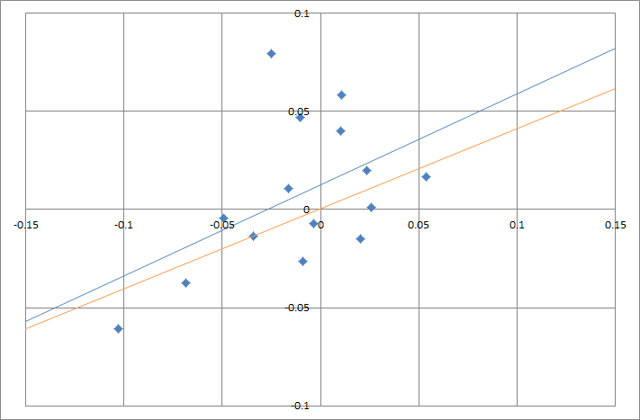In this chart, there are 15 blue points, and the blue line is the conventional linear regression. The x-axis corresponds to the benchmark; the y-axis corresponds to my monthly returns. So the highest point shows that during one month the benchmark lost 2.5% while my portfolio gained 7.9%, and the lowest point shows that during one month the benchmark lost 10.3% while my portfolio lost 6.1%. This blue line gets as close to the 15 points as possible if you measure closeness by the square of the vertical distance between the line and each point and add them all together. This regression method is thus called "ordinary least squares," or OLS for short, and it's been in use for over 200 years. Beta is the slope of the line (in this case 0.46) and alpha is where the line crosses the y axis (in this case 1.3% monthly, or 16.3% annualized).

The orange line is the alternative regression line calculated by Theil and Sen's method. Notice that there are six points above the OLS line and nine points below it. But the TS line (Theil and Sen line) leaves seven points above it and seven points below it, and it intersects one point. TS regression lines, which are calculated by using medians, always leave just as many points above and below them. Neither line fits the data very well, but to my eyes, at least the orange line seems like a better fit.

To get this line, you go through two steps. First, you calculate beta by taking all possible slopes between pairs of points and getting the median. With 15 points, that means calculating 105 different slopes. The median of all of them turns out to be 0.41. Second, you calculate alpha, or the y-intercept, by taking the y values, subtracting beta times the x values, and taking the median of all of those. Here, the TS alpha is only 0.6% annualized.

Among the few who have examined the subject, the scientific consensus appears to be that no matter what the data is, TS regression is superior to OLS regression. There's an excellent paper about this called "Linear Valuation Without OLS: The Theil-Sen Estimation Approach." In it, the authors cite studies that prove that TS regression not only gets rid of outliers, which tend to have an outsized effect on OLS regression, but also take care of the problem of heteroscedasticity. (Homoscedasticity means that the variance of the data is evenly distributed over the independent variables and heteroscedasticity means that it isn't.) Basically, OLS regression only works well when the data is more or less homoscedastic, and if you've ever looked at a typical scatter chart for monthly returns, you'll see that the dots tend to coalesce around the middle (see the first chart in this article for a good example).

The authors don't apply TS regression to the calculation of alpha in this paper, instead applying it to valuation multiples, with striking results. As far as I know, nobody has yet proposed using TS regression to calculate alpha. But it can be done.

## OLS vs TS

A large majority of the time, OLS and TS alpha and beta are very close. But there are some significant exceptions.

Let's look at some ETFs as examples. The chart below shows the last three years' performance of the iShares MSCI Brazil Small-Cap ETF (NASDAQ:EWZS) compared to the Vanguard Total Stock Market ETF (NYSEARCA:VTI). As before, the blue line is the OLS regression line and the orange line is the TS regression line.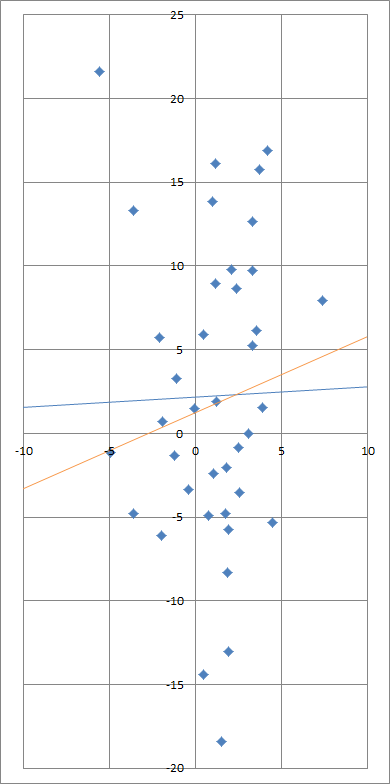EWZS's total return was 27% compared to VTI's 14%. Its annualized TS alpha is 17% while its annualized OLS alpha is 27%. Its TS beta is 0.45 while its OLS beta is 0.07. The OLS beta is so low due to one outlier (-5.6, 21.6). Without that piece of data, the two lines would be almost exactly the same.

Now let's take a look at the actual chart.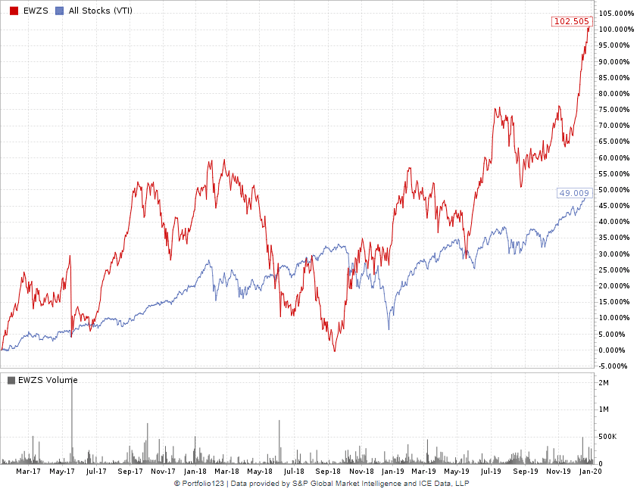Does this look like a stock with a beta close to 0, a stock with absolutely no correlation to the market? If you look at the last year alone, its OLS beta is 0.79, and if you look at the first of the three years, its OLS beta is 0.75. Why should its performance in 2018 (in particular, one month in 2018) give it a three-year beta of 0.07? Does EWZS look like it deserves an annualized alpha of 27%?

Now let's compare this with another ETF, the iShares PHLX Semiconductor ETF (NASDAQ:SOXX).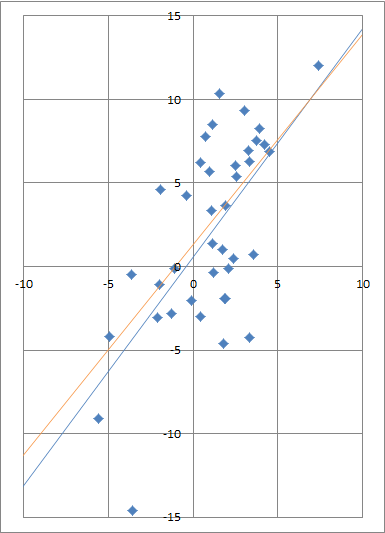SOXX's annualized return over the last three years is 28%, quite close to that of EWZS. Here, the annualized TS alpha is 18% while the annualized OLS alpha is only 7%. The TS beta is 1.26 while the OLS beta is 1.37. Once again, an outlier (-3.6, -14.6) makes all the difference-take that outlier away, and the lines are almost the same.

Here's the chart: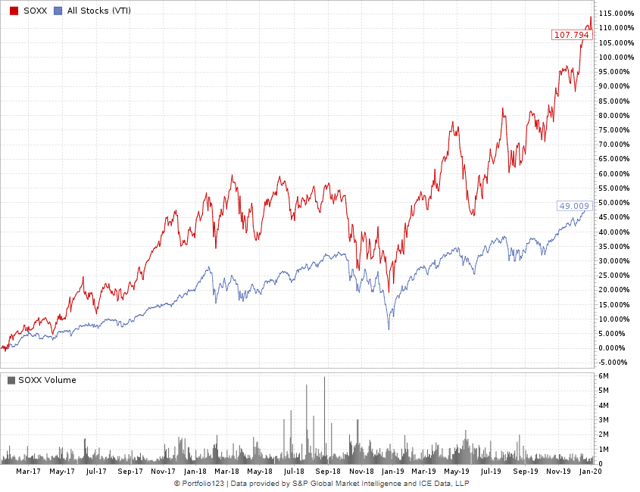Looking at the charts of EWZS and SOXX, it looks like they deserve about the same alpha. And indeed, by the TS measure, they have the same alpha. By OLS measures, however, EWZS has an alpha of 27% while SOXX has an alpha of only 7%.

## Application and Calculation of TS Alpha

Because I view alpha as a superior performance measure to CAGR, to measures based on average returns (e.g. the Sharpe ratio), and to measures based on excess returns, I use alpha when I backtest portfolio strategies (I use www.portfolio123.com for all my data and backtesting needs). And because I view TS alpha as superior to OLS alpha, I've been relying primarily on that measure to tell me when one strategy is superior to another.

You can easily calculate the Theil-Sen slope (beta) in R, MATLAB, and Python (in Excel, it's significantly more difficult). After you get TS beta, you take each of the portfolio returns and subtract the product of the TS slope and the benchmark return, you then calculate the median of those numbers. That is TS alpha.

If you'd rather use Excel than R, MATLAB, or Python, I've created an Excel file here that calculates TS alpha and beta from forty returns. You can see pretty easily how it's done, and then adapt it for the number of returns you have.

No matter which program you use, the number of calculations required will be about half the square of the number of returns. So if you have thousands of returns, I recommend taking a sample of the slopes rather than calculating all of them.

## Conclusion

Admittedly, I picked some rather extreme examples above. In the majority of cases, OLS and TS alpha aren't terribly different from one another. In the examples, TS alpha was closer to the excess returns than OLS alpha, but this is not representative; TS alpha is very often farther. Both can be valuable measures of portfolio performance. Theoretically, however, TS alpha is a more reliable measure and tends to the fit the data better.

OLS measures dominate the world of finance. Standard deviation is an OLS measure, which makes the Sharpe ratio and the information ratio OLS measures as well. T-tests and p-tests are OLS measures, and so is R-squared. Some statisticians have been appalled by the proclivity of the world of finance to apply OLS measures to financial and economic data, with its heteroscedasticity and its fat tails. But these measures have persisted because they're easy to work with and have been used for centuries.

James Ohlson and Seil Kim, the authors of "Linear Valuation without OLS," wrote, "consider the following counter-factual scenario. Suppose the history of empirical accounting [or financial] research had never been aware of OLS and instead had picked up on TS and used it as a standard paradigm. Now suppose that someone suddenly discovers OLS and tries to sell this new approach to the research community. Would that be an easy sell with plenty of takers? Not likely. What would be the arguments?"

It would be nice if we could slowly replace OLS measures with measures that are not dependent on normally distributed data, measures that are insensitive to outliers. Perhaps using Theil-Sen estimation for alpha and beta - and using Kendall's tau, a very closely related measure, for correlation - would be a good start. But I don't expect to see it happening anytime soon.

Disclosure: I/we have no positions in any stocks mentioned, and no plans to initiate any positions within the next 72 hours. I wrote this article myself, and it expresses my own opinions. I am not receiving compensation for it. I have no business relationship with any company whose stock is mentioned in this article.